# NEEP 541 Sputtering Fall 2002 Jake Blanchard Outline

• Slides: 24
Download presentationNEEP 541 – Sputtering Fall 2002 Jake BlanchardOutline n Sputtering n n n Definitions Models Sample Data Angle of Incidence Correlations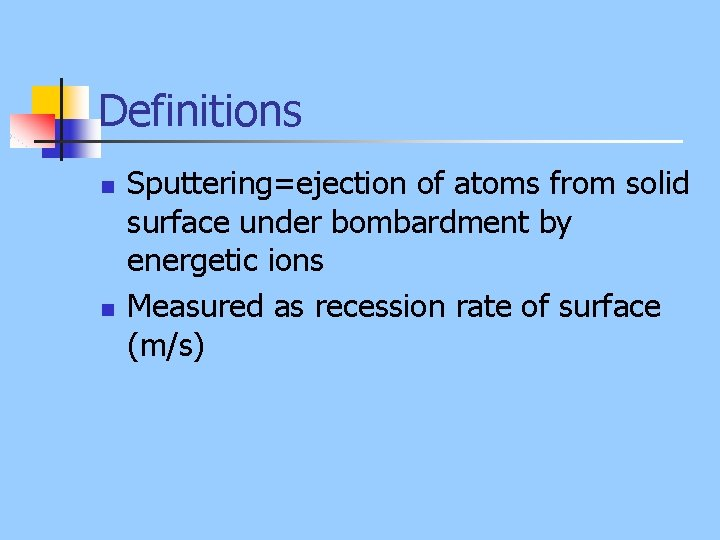Definitions n n Sputtering=ejection of atoms from solid surface under bombardment by energetic ions Measured as recession rate of surface (m/s)Schematic of Process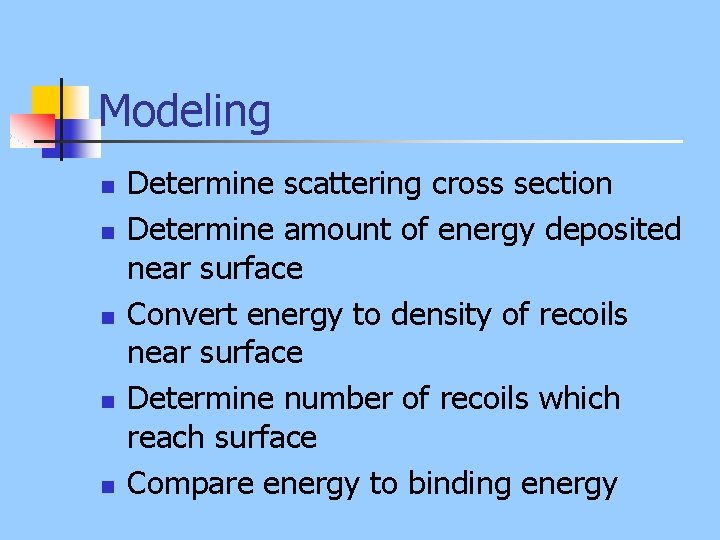Modeling n n n Determine scattering cross section Determine amount of energy deposited near surface Convert energy to density of recoils near surface Determine number of recoils which reach surface Compare energy to binding energy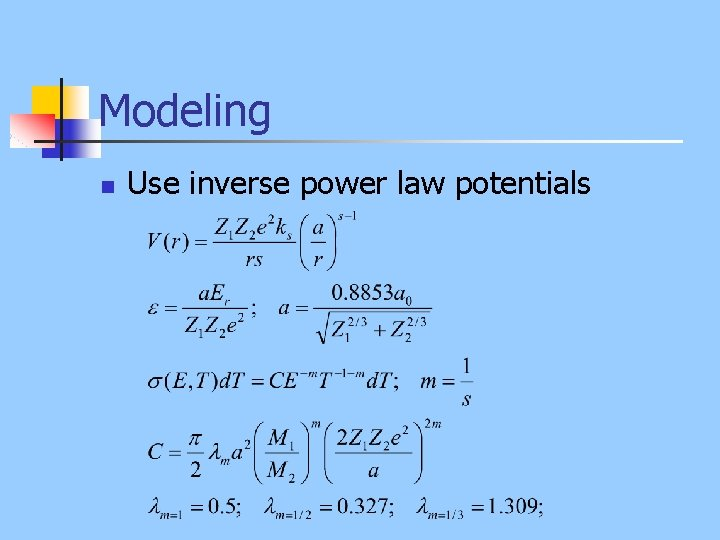Modeling n Use inverse power law potentials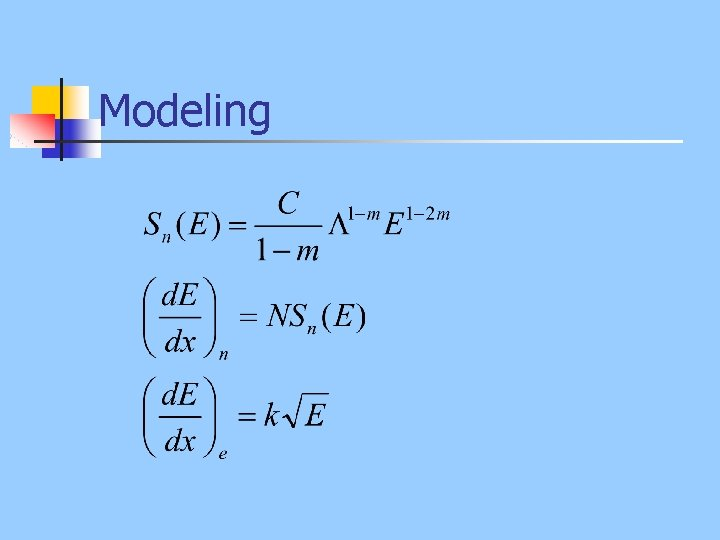ModelingSigmund Theory n n Assume sputtering is proportional to energy deposited (FD) near surface Hence, sputtering is proportional to stopping power near surface Angle of incidenceStopping and Sputtering Distributions – Kr on Cu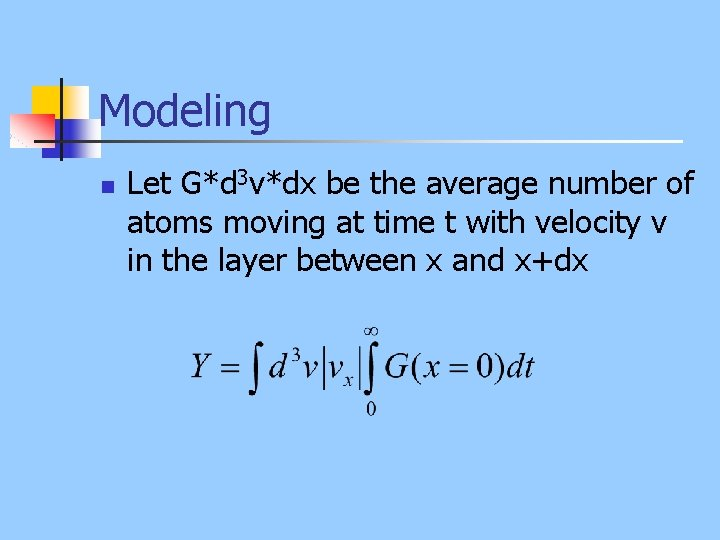Modeling n Let G*d 3 v*dx be the average number of atoms moving at time t with velocity v in the layer between x and x+dx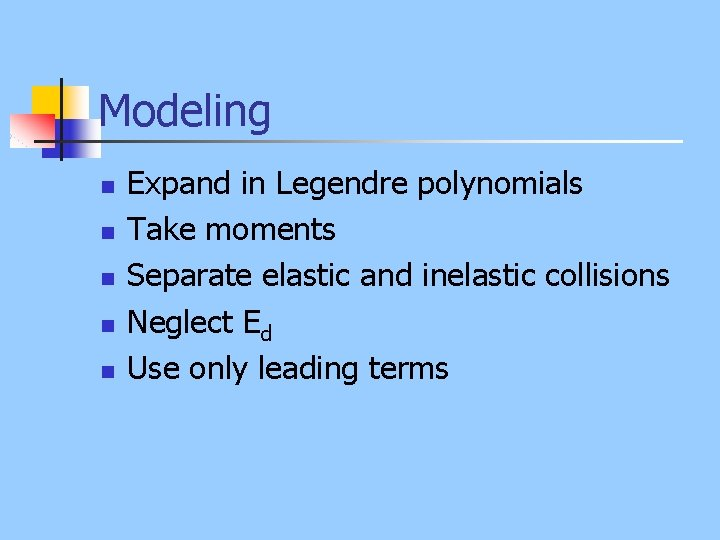Modeling n n n Expand in Legendre polynomials Take moments Separate elastic and inelastic collisions Neglect Ed Use only leading termsResult n depends on m, incidence angle, and on mass ratio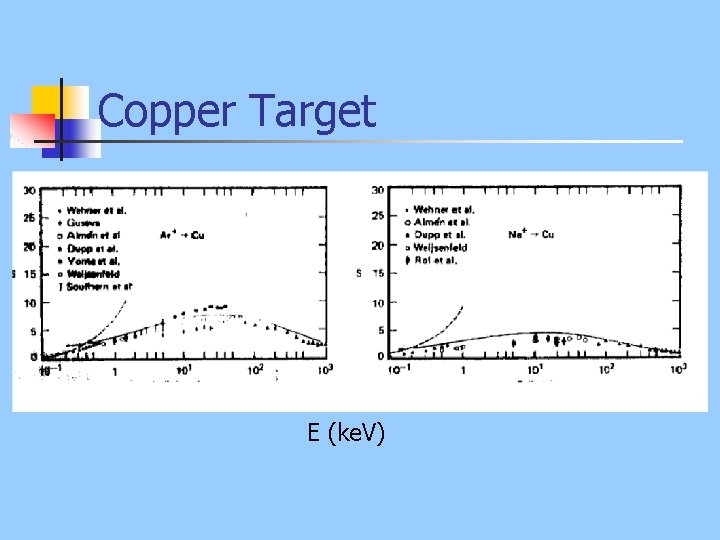Copper Target E (ke. V)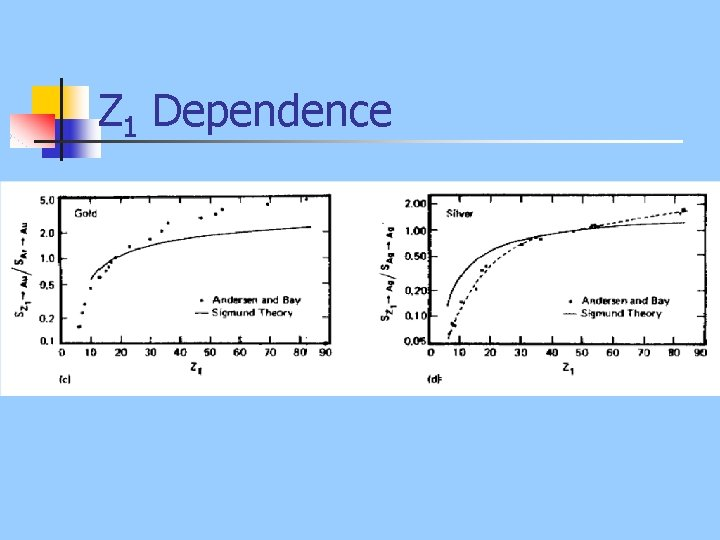Z 1 DependenceHe on ? ?Tungsten Self-SputteringMass Ratio DependenceAngle of Incidence n Empirically, the dependence of sputtering on the angle of incidence is: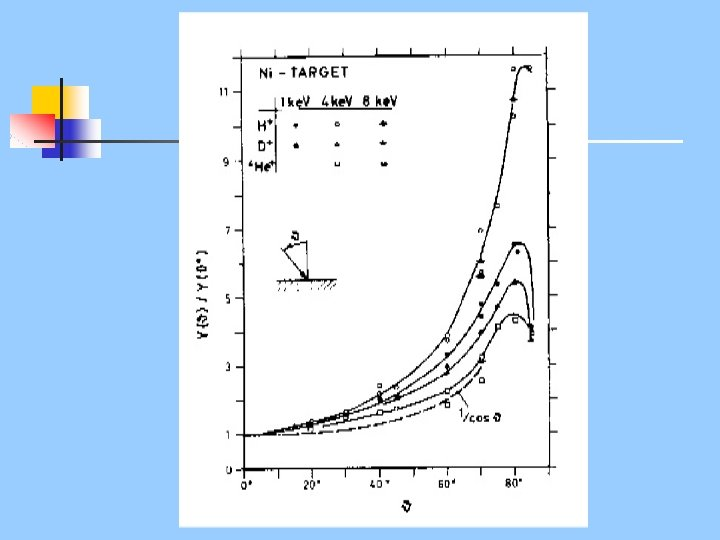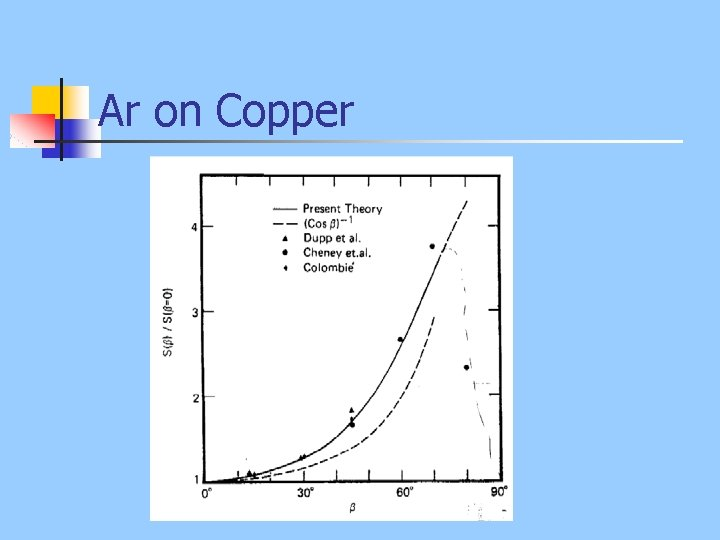Ar on Copper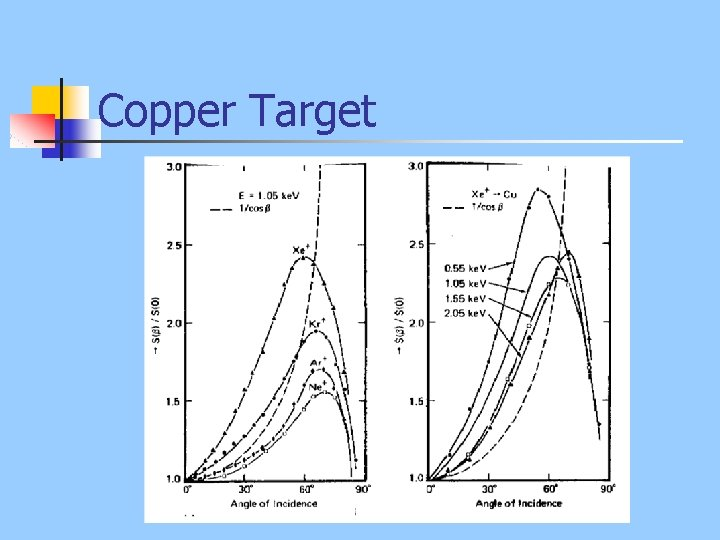Copper TargetEmpirical Correlation threshold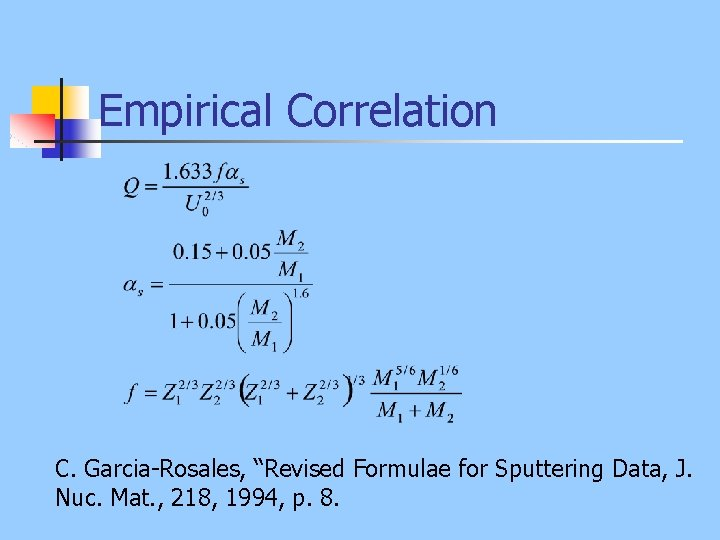Empirical Correlation C. Garcia-Rosales, “Revised Formulae for Sputtering Data, J. Nuc. Mat. , 218, 1994, p. 8.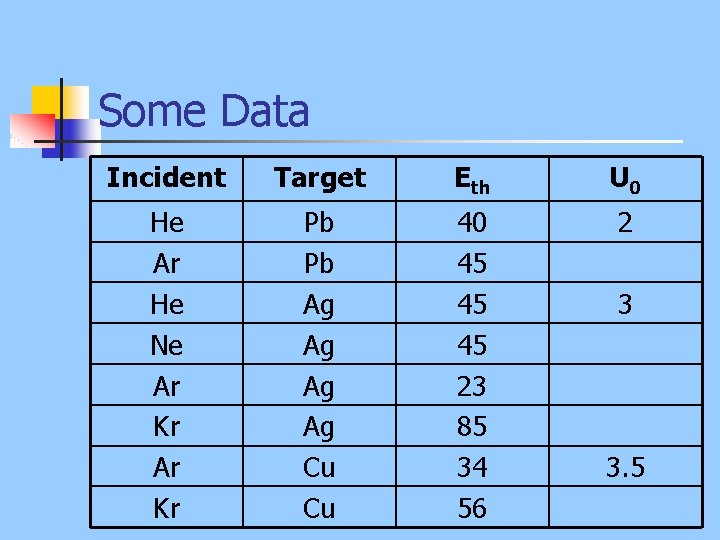Some Data Incident Target Eth U 0 He Ar He Ne Ar Kr Pb Pb Ag Ag Cu Cu 40 45 45 45 23 85 34 56 2 3 3. 5